# GMAT Math : DSQ: Calculating the slope of parallel lines

## Example Questions

### Example Question #1 : Dsq: Calculating The Slope Of Parallel Lines

Consider this system of equations: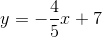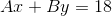Does this system has exactly one solution?

Statement 1: The lines representing the equations are parallel.

Statement 2: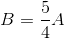BOTH statements TOGETHER are insufficient to answer the question.

EITHER statement ALONE is sufficient to answer the question.

Statement 1 ALONE is sufficient to answer the question, but Statement 2 ALONE is NOT sufficient to answer the question.

BOTH statements TOGETHER are sufficient to answer the question, but NEITHER statement ALONE is sufficient to answer the question.

Statement 2 ALONE is sufficient to answer the question, but Statement 1 ALONE is NOT sufficient to answer the question.

EITHER statement ALONE is sufficient to answer the question.

Explanation:

The solution of a system of linear equations is the point at which their lines intersect; if they are parallel, then by definition, there is no such point, and the system has no solution.

If, then we can rewrite the second equation as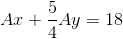In slope-intercept form: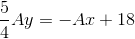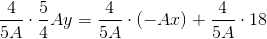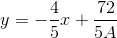This line has a slope of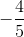. The other equation has a line with slope ofalso, as can be easily seen since it is already in slope-intercept form. Since both equations have lines with the same slope, they are either the same line or parallel lines; either way, the system does not have exactly one solution.

### Example Question #2 : Dsq: Calculating The Slope Of Parallel Lines

If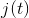is modeled by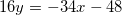, find the slope of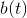.

I)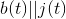.

II)crosses the-axis at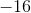.

Statement I is sufficient to answer the question, but Statement II is not sufficient to answer the question.

Statement II is sufficient to answer the question, but Statement I is not sufficient to answer the question.

Either statement alone is sufficient to answer the question.

Both statements together are needed to answer the question.

Statement I is sufficient to answer the question, but Statement II is not sufficient to answer the question.

Explanation:

I) Tells us the two lines are parallel. Parallel lines have the same slope.

II) Gives us the x-intercept of b(t). By itself this gives us no clue as to the slop of b. If we had another point on b(t) we could find the slope, but we don't have another point.

So, statement I is what we need.

### Example Question #3 : Dsq: Calculating The Slope Of Parallel Lines

Calculate the slope of a line parallel to line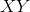.

1. Linepasses through points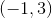and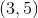.
2. The equation for lineis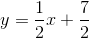.

Each statement alone is sufficient to answer the question.

Both statements taken together are sufficient to answer the question, but neither statement alone is sufficient.

Statement 2 alone is sufficient, but statement 1 alone is not sufficient to answer the question.

Statement 1 alone is sufficient, but statement 2 alone is not sufficient to answer the question.

Statements 1 and 2 are not sufficient, and additional data is needed to answer the question.

Each statement alone is sufficient to answer the question.

Explanation:

Statement 1: Since we're referring to a line parallel to line XY, the slopes will be identical. We can use the points provided to calculate the slope: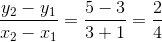We can simplify the slope to just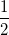.

Statement 2: Finding the slope of a line parallel to line XY is really straightforward when given the equation of a line.Whereis the slope andthe y-intercept.

In this case, ourvalue is.\

Each statement alone is sufficient to answer the question.

### Example Question #4 : Dsq: Calculating The Slope Of Parallel Lines

Find the slope of the line parallel to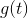.

I)passes through the point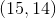.

II)has an x-intercept of 290.

Statement I is sufficient to answer the question, but Statement II is not sufficient to answer the question.

Either statement is sufficient to answer the question.

Both statements are needed to answer the question.

Statement II is sufficient to answer the question, but Statement I is not sufficient to answer the question.

Both statements are needed to answer the question.

Explanation:

Recall that parallel lines have the same slope and that slope can be calculated from any two points.

Statement I gives us a point onStatement II gives us the x-intercept, a.k.a. the point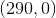.

Therfore, using both statements, we can find the slope ofand any line parallel to it.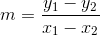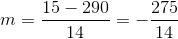Tired of practice problems?

Try live online GMAT prep today.